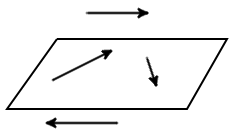Study of mathematics online.
Study math with us and make sure that "Mathematics is easy!"

# Vectors Definition. Main information

## Vector definition

Definition. Vector is a directed line segment, ie the segment having a length and a definite direction. Graphically vector depicted as a directed line segments of a certain length. (Fig. 1)Fig. 1

## Vector designation

The vector which has a beginning point A and end point B, denoted AB (Fig. 1). Also, the vector represent one small letter, for example a.a.

## Vector length

Definition. The length of the directed segment determines the numerical value of the vector and is called the length of the vector AB.

The length of the vector AB is denoted as: |AB|.

## Zero vector

Definition. Zero vector is a vector whose start and end points coincide.

The zero vector is usually is denoted as 0.

The length of the zero vector is zero.

## Collinear vectors

Definition. Vector parallel to one line or lying on one line are called collinear vectors (Fig. 2).Fig. 2

## Codirected vectors

Definition. Two collinear vectors a and b are called codirected vectors if their directions are the same: a↑↑b (Fig. 3).Fig. 3

## Oppositely directed vectors

Definition. Two collinear vectors a and b are called oppositely directed vectors if their directions are opposite: a↑↓b (Fig. 4).Fig. 4

## Coplanar vectors

Definition. Vectors parallel to the same plane, or lie on the same plane are called coplanar vectors (Fig. 5).Fig. 5

It is always possible to find a plane parallel to the two random vectors, in that any two vectors are always coplanar.

## Equal vectors

Definition. Vectors a and b is an equal vectors if they are in the same or parallel lines, their directions are the same and the lengths are equal (Fig. 6).Fig. 6

Two vectors are equal if they are collinear, codirected and have the same length:

a = b, if a↑↑b and |a| = |b|.

## Unit vector

Definition. Unit vector or orth is a vector whose length is equal to one.